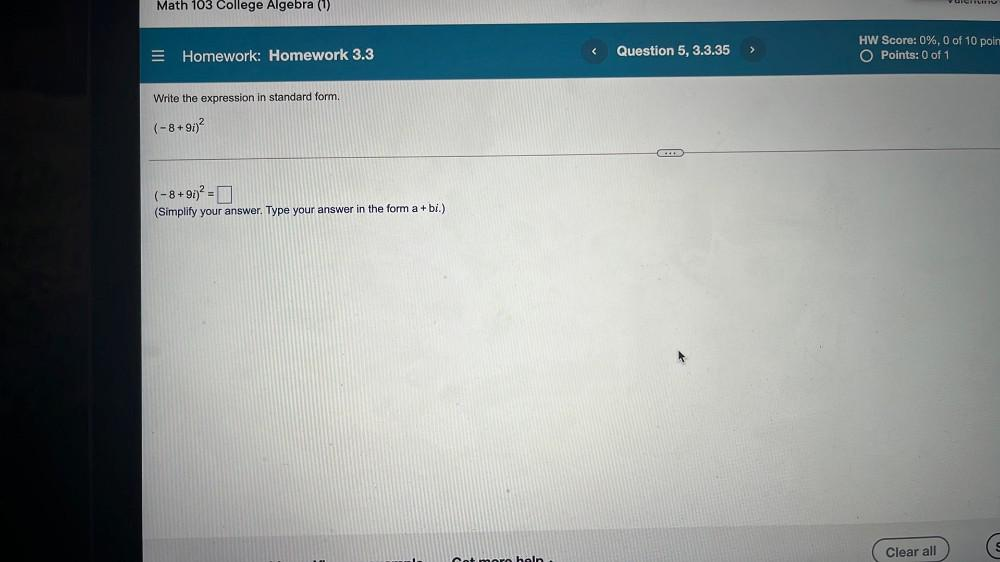Question:

# VUCITLITIU Math 103 College Algebra (1) s > Question 5, 3.3.35 = Homework: Homework 3.3 HW Score: 0%, 0 of 10 poin O Points: 0 oVUCITLITIU Math 103 College Algebra (1) s > Question 5, 3.3.35 = Homework: Homework 3.3 HW Score: 0%, 0 of 10 poin O Points: 0 of 1 Write the expression in standard form. (-8+90)2 ... (-8+91)2 = 0 (Simplify your answer. Type your answer in the form a + bi.) Clear all